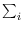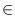Next: Memory Management Up: Simple Data Types and Previous: Random Variates ( random_variate   Contents   Index

# Dynamic Random Variates ( dynamic_random_variate )

Definition

An instance R of the data type dynamic_random_variate is a non-uniform random number generator. The generation process is governed by an array<int> w. Let [l..r] be the index range of w and let W =w[i] be the total weight. Then any integer i[l..h] is generated with probability w[i]/W. The weight function w must be non-negative and W must be non-zero. The weight function can be changed dynamically.

#include < LEDA/core/random_variate.h >

Creation

 dynamic_random_variate R(const array& w) creates an instance R of type dynamic_random_variate.

Operations

 int R.generate() generates i[l..h] with probability w[i]/W. int R.set_weight(int i, int g) sets w[i] to g and returns the old value of w[i]. Precondition i[l..h].